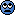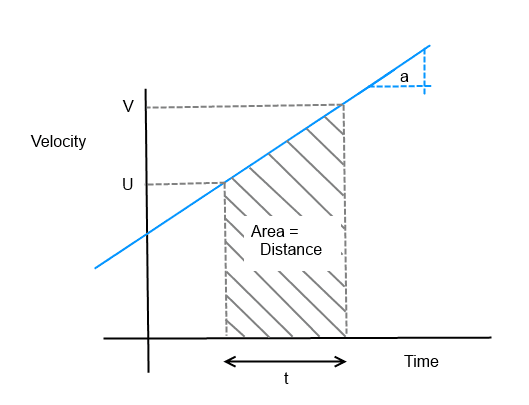# Projectile Motion Kinematics: Finding maximum height

• DracoMalfoy
The SUVAT equations for linear motion under constant acceleration are named after the five variables: s=distance, u=initial speed, v=final speed, a=acceleration, t=time.Any three will determne the remaining two, so there are five equations, each omitting one variable.The trick, then, is to identify the four variables of interest and use the equation involving those. In the present case it was s, u, v and a. Note that this particular equation is equivalent to the work conservation equation for constant force:W=F/Af

## Homework Statement

A ball is kicked with an initial speed of 20m/s at an angle of 50° above the horizontal. What maximum height does the ball reach?

## Homework Equations

• Vfx=VicosΘ
• Δx=VicosΘt
• Vfy=(VisinΘ)+ay⋅t
• (Vfy)^2=(VisinΘ)^2+2ay⋅Δy
• Δy=1/2(Vfy+(VisinΘ))⋅t

## The Attempt at a Solution

I know that the final velocity for both x and y is 20m/s if that is the case. The acceleration for the x-direction is 0m/s^2 while its -9.8m/s^2 due to Earth gravitational acceleration. Vy at max height is 0.

Known
• Vfx and Vfy= 20m/s
• Θ=50°
• Vfy=0m/s
• ax=0m/s^2
• ay=-9.8m/s^2
• T=?
• Δy=?
• Δx=?

I'm assuming that the question is asking for max height as Δy in the y-direction. I was trying to solve for time in order to use the equation to solve for Δy but I can't see how to do that. I'm not sure if there is another way that I could do this or if I just labeled things incorrectly.

## Homework Statement

A ball is kicked with an initial speed of 20m/s at an angle of 50° above the horizontal. What maximum height does the ball reach?

## Homework Equations

• Vfx=VicosΘ
• Δx=VicosΘt
• Vfy=(VisinΘ)+ay⋅t
• (Vfy)^2=(VisinΘ)^2+2ay⋅Δy
• Δy=1/2(Vfy+(VisinΘ))⋅t

## The Attempt at a Solution

I know that the final velocity for both x and y is 20m/s if that is the case. The acceleration for the x-direction is 0m/s^2 while its -9.8m/s^2 due to Earth gravitational acceleration. Vy at max height is 0.

Known
• Vfx and Vfy= 20m/s
• Θ=50°
• Vfy=0m/s
• ax=0m/s^2
• ay=-9.8m/s^2
• T=?
• Δy=?
• Δx=?

I'm assuming that the question is asking for max height as Δy in the y-direction. I was trying to solve for time in order to use the equation to solve for Δy but I can't see how to do that. I'm not sure if there is another way that I could do this or if I just labeled things incorrectly.

You do not need to "assume" anything about what the question is asking---it tells you exactly what it wants.

Anyway, the initial speed is ##v_0 = 20## m/s, but at an angle of 50° up. From that, you need to figure out the x- and y-components ##v_{0x}## and ##v_{0y}##. They are NOT 20 m/s: draw a picture to see why.

After you have found ##v_{0x}## and ##v_{0y}## the rest of the question ought to be fairly straightforward..

I found the x and y components. I am a bit confused now..

Why are you using an equation to find the time? You have a much more useful equation in terms of squared initial and final velocities, acceleration and distance: V_f^2 = V_i^2 + 2ad. You wrote down the expression for the initial vertical velocity and you said you know the final vertical velocity is zero at the peak of its flight. You know the acceleration.

Why are you using an equation to find the time? You have a much more useful equation in terms of squared initial and final velocities, acceleration and distance: V_f^2 = V_i^2 + 2ad. You wrote down the expression for the initial vertical velocity and you said you know the final vertical velocity is zero at the peak of its flight. You know the acceleration.
i tried doing it that way but i didnt get the answer. i don't know if i labeled things correctly.

I found the x and y components. I am a bit confused now..
So what did you get for the y component?

So what did you get for the y component?
15.3is this correct. I solved for t using
• Vfy=(VisinΘ)+ay⋅t
i got 1.56s

I then used

• Δy=1/2(Vfy+(VisinΘ))⋅t
and got 11.9m or 12m

That looks correct to me, however you could do it in one step if you used another of the SUVAT equations...

V2 = U2 + 2as

That looks correct to me, however you could do it in one step if you used another of the SUVAT equations...

V2 = U2 + 2as
I see :| my teacher gave us like 8 equations to memorize... I have no clue of any of the other methods

I see :| my teacher gave us like 8 equations to memorize... I have no clue of any of the other methods
The SUVAT equations for linear motion under constant acceleration are named after the five variables: s=distance, u=initial speed, v=final speed, a=acceleration, t=time.
Any three will determne the remaining two, so there are five equations, each omitting one variable.
The trick, then, is to identify the four variables of interest and use the equation involving those.
In the present case it was s, u, v and a. Note that this particular equation is equivalent to the work conservation equation for constant force: ΔKE=F.d.

Note that this particular equation is equivalent to the work conservation equation for constant force: ΔKE=F.d.

If you are also studying physics and have covered conservation of energy then this is how you get there...

Conservation of energy says..
Final Kinetic Energy = Initial Kinetic Energy + work done on object
0.5mV2 = 0.5mU2 + ma*s

multiply both sides by 2
mV2 = mU2 + m2as

mass cancels..
V2 = U2 + 2as

my teacher gave us like 8 equations to memorize
Thing to do is understand what's going on. That way you don't need to memorize so much, or, indeed, nothing at all.

+1

There are ways to understand them, for example..

S = (U+V)t/2

...is the average velocity (V+U)/2, multiplied by time t.

You can also think of it as the area under a graph of velocity vs time.## Homework Statement

A ball is kicked with an initial speed of 20m/s at an angle of 50° above the horizontal. What maximum height does the ball reach?

## Homework Equations

• Vfx=VicosΘ
• Δx=VicosΘt
• Vfy=(VisinΘ)+ay⋅t
• (Vfy)^2=(VisinΘ)^2+2ay⋅Δy
• Δy=1/2(Vfy+(VisinΘ))⋅t

## The Attempt at a Solution

I know that the final velocity for both x and y is 20m/s if that is the case. The acceleration for the x-direction is 0m/s^2 while its -9.8m/s^2 due to Earth gravitational acceleration. Vy at max height is 0.

Known
• Vfx and Vfy= 20m/s
• Θ=50°
• Vfy=0m/s
• ax=0m/s^2
• ay=-9.8m/s^2
• T=?
• Δy=?
• Δx=?

I'm assuming that the question is asking for max height as Δy in the y-direction. I was trying to solve for time in order to use the equation to solve for Δy but I can't see how to do that. I'm not sure if there is another way that I could do this or if I just labeled things incorrectly.

This is fundamentally the same as your "Stone thrown from a bridge" question you posted. Solve one of them and you should see how to solve them all. You need to do some reading up on projectile motion.

•davenn